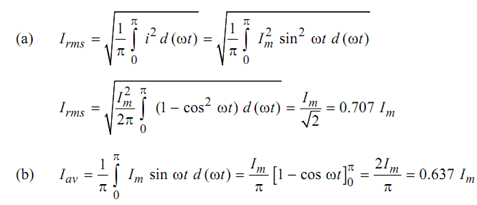## Find out form factor and peak factor, Electrical Engineering

Assignment Help:

Find out Form factor and Peak factor:

For the output of full wave rectifier, find out

(a) RMS value,

(b) Average value,

(c) Form factor, and

(d) Peak factor.

Solution

The output current of full wave rectifier

Time period = π(c) Form factor K f  = Irms / Iav  =0.707 Im/0.637 Im

(d) Peak factor K =  Im/ Irms  = Im /0.707 Im= 1.414

#### Find the diameter of a circular waveguide, Q Find the diameter of a circula...

Q Find the diameter of a circular waveguide that will have a lower cutoff frequency of 10 GHz and also specify its largest usable frequency.

#### Network theorem, what is couple circuit ?

what is couple circuit ?

#### Can you draw robot configuration, a) Draw robot configuration. b) Explai...

a) Draw robot configuration. b) Explain the significance of end effectors. c) Define the terms (i) accuracy (ii) Repeatability (iii) Work volume (iv) Control Reso

#### What is piconet, A collection of devices connected by Bluetooth technology ...

A collection of devices connected by Bluetooth technology in an ad hoc fashion.

#### Draw the energy diagram of a pn junction, Question: a). Draw the energy...

Question: a). Draw the energy diagram of a pn junction: (i) at thermal equilibrium, (ii) when a forward bias with magnitude half the built in potential (V = φk/2) is applie

#### Show the load current is independent of zl, Q. A negative impedance convert...

Q. A negative impedance converter is used, as shown in Figure. Show that the load current i L is given by v in /R, which is independent of Z L . Note that since the load sees a cu

#### Ral rotate accumulator left through carry instruction , RAL Rotate Accumula...

RAL Rotate Accumulator Left Through Carry Instruction This  instruction rotates the content  of the accumulator  towards left by one bit. The D 0 bit moves to D 1   bit moves

#### Measurement of frequency by wein''s bridge, Q.  Explain the measurement of...

Q.  Explain the measurement of frequency in detail by using Wein's bridge. Sol. The Wein's bridge is presented here not only for its use as an AC bridge to measure frequency

#### Find the duty ratio and neglecting friction and windage, A 440-V, 60-Hz, si...

A 440-V, 60-Hz, six-pole, wye-connected, wound-rotor induction motor with a full-load speed of 1170 r/min has the following per-phase parameters referred to the stator:R 1 = R' 2

#### Find the new gain for the inverting-amplifier circuit, Q. An op amp has a f...

Q. An op amp has a finite gain of only 50, but is otherwise ideal. For the inverting-amplifier circuit of Figure, if R 2 = 20 k, what value of R 1 would be needed to give a gain